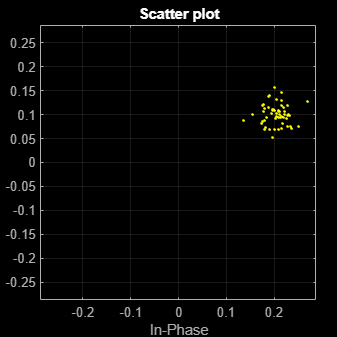# wlanHTLTFChannelEstimate

Channel estimation using HT-LTF

## Syntax

``chEst = wlanHTLTFChannelEstimate(demodSig,cfg)``
``chEst = wlanHTLTFChannelEstimate(demodSig,cfg,span)``

## Description

example

````chEst = wlanHTLTFChannelEstimate(demodSig,cfg)` returns the channel estimate using the demodulated HT-LTF1 signal, `demodSig`, given the parameters specified in configuration object `cfg`.```

example

````chEst = wlanHTLTFChannelEstimate(demodSig,cfg,span)` returns the channel estimate and specifies the span of a moving-average filter used to perform frequency smoothing.```

## Examples

collapse all

Estimate and plot the channel coefficients of an HT-mixed format channel by using the high throughput long training field.

Create an HT format configuration object. Generate the corresponding HT-LTF based on the object.

```cfg = wlanHTConfig; txSig = wlanHTLTF(cfg);```

Multiply the transmitted HT-LTF signal by 0.2 + 0.1i and pass it through an AWGN channel. Demodulate the received signal.

```rxSig = awgn(txSig*(0.2+0.1i),30); demodSig = wlanHTLTFDemodulate(rxSig,cfg);```

Estimate the channel response using the demodulated HT-LTF.

`est = wlanHTLTFChannelEstimate(demodSig,cfg);`

Plot the channel estimate.

```scatterplot(est) grid```The channel estimate matches the complex channel multiplier.

Estimate the channel coefficients of a 2x2 MIMO channel by using the high throughput long training field. Recover the HT-data field and determine the number of bit errors.

Create an HT-mixed format configuration object for a channel having two spatial streams and four transmit antennas. Transmit a complete HT waveform.

```cfg = wlanHTConfig('NumTransmitAntennas',2, ... 'NumSpaceTimeStreams',2,'MCS',11); txPSDU = randi([0 1],8*cfg.PSDULength,1); txWaveform = wlanWaveformGenerator(txPSDU,cfg);```

Pass the transmitted waveform through a 2x2 TGn channel.

```tgnChan = wlanTGnChannel('SampleRate',20e6, ... 'NumTransmitAntennas',2, ... 'NumReceiveAntennas',2, ... 'LargeScaleFadingEffect','Pathloss and shadowing'); rxWaveformNoNoise = tgnChan(txWaveform);```

Create an AWGN channel with noise power, `nVar`, corresponding to a receiver having a 9 dB noise figure. The noise power is equal to kTBF, where k is Boltzmann's constant, T is the ambient noise temperature (290K), B is the bandwidth (20 MHz), and F is the noise figure (9 dB).

```nVar = 10^((-228.6 + 10*log10(290) + 10*log10(20e6) + 9)/10); awgnChan = comm.AWGNChannel('NoiseMethod','Variance', ... 'Variance',nVar);```

Pass the signal through the AWGN channel.

`rxWaveform = awgnChan(rxWaveformNoNoise);`

Determine the indices for the HT-LTF. Extract the HT-LTF from the received waveform. Demodulate the HT-LTF.

```indLTF = wlanFieldIndices(cfg,'HT-LTF'); rxLTF = rxWaveform(indLTF(1):indLTF(2),:); ltfDemodSig = wlanHTLTFDemodulate(rxLTF,cfg);```

Generate the channel estimate by using the demodulated HT-LTF signal. Specify a smoothing filter span of three subcarriers.

`chEst = wlanHTLTFChannelEstimate(ltfDemodSig,cfg,3);`

Extract the HT-data field from the received waveform.

```indData = wlanFieldIndices(cfg,'HT-Data'); rxDataField = rxWaveform(indData(1):indData(2),:);```

Recover the data and verify that there no bit errors occurred.

```rxPSDU = wlanHTDataRecover(rxDataField,chEst,nVar,cfg); numErrs = biterr(txPSDU,rxPSDU)```
```numErrs = 0 ```

## Input Arguments

collapse all

Demodulated HT-LTF signal, specified as an NST-by-NSYM-by-NR array. NST is the number of occupied subcarriers, NSYM is the number of HT-LTF OFDM symbols, and NR is the number of receive antennas.

Data Types: `single` | `double`
Complex Number Support: Yes

Configuration information, specified as a `wlanHTConfig` object.

Filter span of the frequency smoothing filter, specified as a positive odd integer and expressed as a number of subcarriers. Frequency smoothing is applied only when `span` is specified and greater than one. See Frequency Smoothing.

Data Types: `single` | `double`

## Output Arguments

collapse all

Channel estimate between all combinations of space-time streams and receive antennas, returned as an NST-by-(NSTS+NESS)-by-NR array. NST is the number of occupied subcarriers, NSTS is the number of space-time streams. NESS is the number of extension spatial streams. NR is the number of receive antennas. Data and pilot subcarriers are included in the channel estimate.

Data Types: `single` | `double`
Complex Number Support: Yes

collapse all

### HT-LTF

The high throughput long training field (HT-LTF) is located between the HT-STF and data field of an HT-mixed packet.As described in Section 19.3.9.4.6 of IEEE® Std 802.11™-2016, the receiver can use the HT-LTF to estimate the MIMO channel between the set of QAM mapper outputs (or, if STBC is applied, the STBC encoder outputs) and the receive chains. The HT-LTF portion has one or two parts. The first part consists of one, two, or four HT-LTFs that are necessary for demodulation of the HT-Data portion of the PPDU. These HT-LTFs are referred to as HT-DLTFs. The optional second part consists of zero, one, two, or four HT-LTFs that can be used to sound extra spatial dimensions of the MIMO channel not utilized by the HT-Data portion of the PPDU. These HT-LTFs are referred to as HT-ELTFs. Each HT long training symbol is 4 μs. The number of space-time streams and the number of extension streams determines the number of HT-LTF symbols transmitted.

Tables 19-12, 19-13 and 90-14 from IEEE Std 802.11-2012 are reproduced here.

`NSTS` Determination`NHTDLTF` Determination`NHTELTF` Determination

Table 19-12 defines the number of space-time streams (NSTS) based on the number of spatial streams (NSS) from the MCS and the STBC field.

Table 19-13 defines the number of HT-DLTFs required for the NSTS.

Table 19-14 defines the number of HT-ELTFs required for the number of extension spatial streams (NESS). NESS is defined in HT-SIG2.

NSS from MCSSTBC fieldNSTS
`1``0``1`
`1``1``2`
`2``0``2`
`2``1``3`
`2``2``4`
`3``0``3`
`3``1``4`
`4``0``4`

NSTSNHTDLTF
`1``1`
`2``2`
`3``4`
`4``4`

NESSNHTELTF
`0``0`
`1``1`
`2``2`
`3``4`

• NHTLTF = NHTDLTF + NHTELTF ≤ 5.

• NSTS + NESS ≤ 4.

• When NSTS = 3, NESS cannot exceed one.

• If NESS = 1 when NSTS = 3 then NHTLTF = 5.

### Frequency Smoothing

Frequency smoothing can improve channel estimation by averaging out noise.

Frequency smoothing is recommended only for cases in which a single transmit antenna is used. Frequency smoothing consists of applying a moving-average filter that spans multiple adjacent subcarriers. Channel conditions dictate whether frequency smoothing is beneficial.

• If adjacent subcarriers are highly correlated, frequency smoothing results in significant noise reduction.

• In a highly frequency-selective channel, smoothing can degrade the quality of the channel estimate.

 IEEE Std 802.11™-2012 IEEE Standard for Information technology — Telecommunications and information exchange between systems, Local and metropolitan area networks — Specific requirements — Part 11: Wireless LAN Medium Access Control (MAC) and Physical Layer (PHY) Specifications.

 Perahia, E., and R. Stacey. Next Generation Wireless LANs: 802.11n and 802.11ac . 2nd Edition, United Kingdom: Cambridge University Press, 2013.